# orthfilt

Orthogonal wavelet filters

## Syntax

``[LoD,HiD,LoR,HiR] = orthfilt(W)``

## Description

example

````[LoD,HiD,LoR,HiR] = orthfilt(W)` computes the four lowpass and highpass, decomposition and reconstruction filters associated with the scaling filter `W` corresponding to a wavelet.```

## Examples

collapse all

Create a scaling filter associated with the Daubechies `db8` wavelet.

```W = dbwavf("db8"); stem(W) title("Original Scaling Filter")```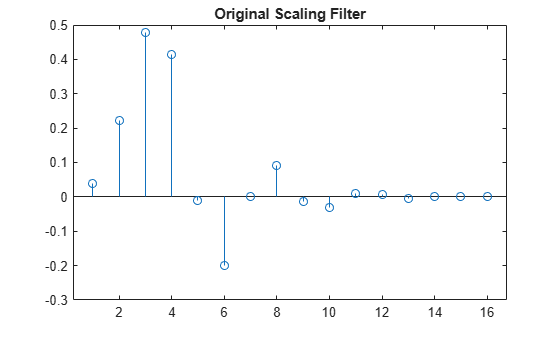Compute the four filters associated with the scaling filter.

`[LoD,HiD,LoR,HiR] = orthfilt(W); `

Plot the decomposition lowpass and highpass filters.

```subplot(2,1,1) stem(LoD) title("Decomposition Lowpass Filter") subplot(2,1,2) stem(HiD) title("Decomposition Highpass Filter")```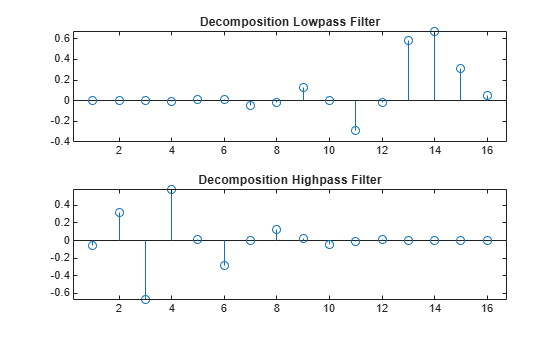Plot the reconstruction lowpass and highpass filters.

```subplot(2,1,1) stem(LoR) title("Reconstruction Lowpass Filter") subplot(2,1,2) stem(HiR) title("Reconstruction Highpass Filter")```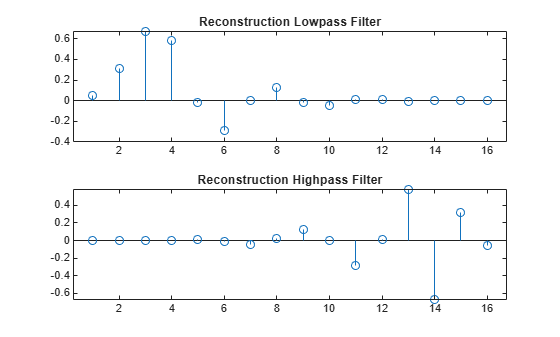Check for orthonormality in the decomposition filters.

```df = [LoD;HiD]; rf = [LoR;HiR]; id = df*df'```
```id = 2×2 1.0000 -0.0000 -0.0000 1.0000 ```

Check for orthonormality in the reconstruction filters.

`id2 = rf*rf'`
```id2 = 2×2 1.0000 0.0000 0.0000 1.0000 ```

Check for orthogonality by dyadic translation.

```df = [LoD 0 0;HiD 0 0]; dft = [0 0 LoD; 0 0 HiD]; zer = df*dft'```
```zer = 2×2 10-12 × -0.1895 -0.0000 0.0000 -0.1895 ```

Plot the low-frequency transfer modulus.

```fftld = fft(LoD); freq = [1:length(LoD)]/length(LoD); figure plot(freq,abs(fftld),"x-") title("Transfer modulus: Lowpass")```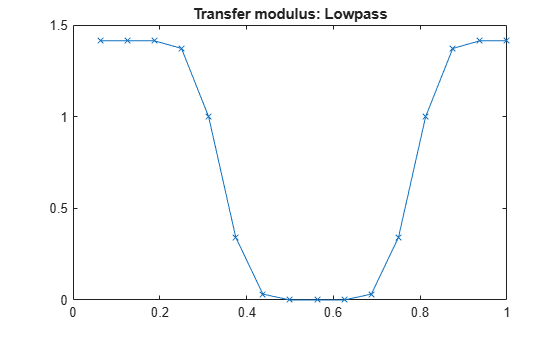Plot the high-frequency transfer modulus.

```ffthd = fft(HiD); plot(freq,abs(ffthd),"x-") title("Transfer modulus: Highpass")```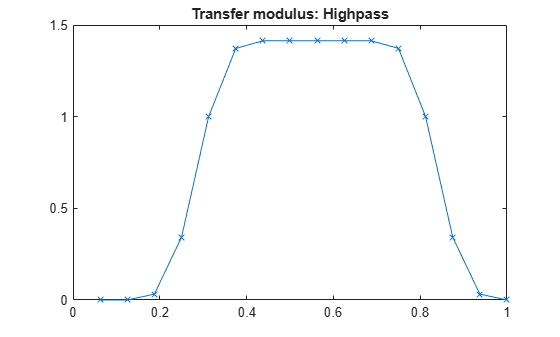## Input Arguments

collapse all

Scaling filter corresponding to a wavelet, specified as a real-valued vector.

## Output Arguments

collapse all

Decomposition lowpass filter associated with the scaling filter `W`, returned as a real-valued vector.

Decomposition highpass filter associated with the scaling filter `W`, returned as a real-valued vector.

Reconstruction lowpass filter associated with the scaling filter `W`, returned as a real-valued vector.

Reconstruction highpass filter associated with the scaling filter `W`, returned as a real-valued vector.

## Algorithms

For an orthogonal wavelet in the multiresolution framework, start with the scaling function ϕ and the wavelet function ψ. One of the fundamental relations is the twin-scale relation:

`$\frac{1}{2}\varphi \left(\frac{x}{2}\right)=\sum _{n\in Z}{w}_{n}\varphi \left(x-n\right)$`

All the filters used in the `dwt` and `idwt` functions are intimately related to the sequence ${\left({w}_{n}\right)}_{n\in Z}$. If ϕ is compactly supported, the sequence (wn) is finite and can be viewed as an FIR filter. The scaling filter `W` is a lowpass FIR filter of length 2N, with the sum 1, and with the norm of 1/√2.

For example, for a `db3` scaling filter,

```w = dbwavf("db3") w = 0.2352 0.5706 0.3252 -0.0955 -0.0604 0.0249 sum(w) = 1.000 norm(w) = 0.7071 ```

Define four FIR filters from filter `W` of length 2N and norm 1.

Filters

Low-Pass

High-Pass

Decomposition

`LoD``HiD`

Reconstruction

`LoR``HiR`

The function computes the four filters using the following scheme.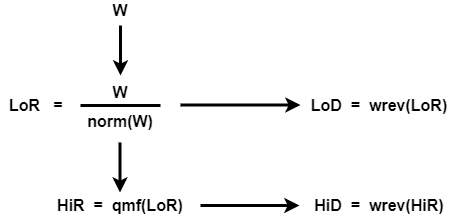`HiR` and `LoR` are quadrature mirror filters: `HiR(k) = (-1)k````LoR(2N + 1 - k)```, for `k = 1, 2, … , 2N`. Because `wrev` reverses vectors, `HiD` and `LoD` are also quadrature mirror filters.

 Daubechies, Ingrid. Ten Lectures on Wavelets. CBMS-NSF Regional Conference Series in Applied Mathematics 61. Philadelphia, Pa: Society for Industrial and Applied Mathematics, 1992.